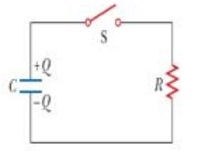# A charged capacitor is connected to a resistor and switch as in the figure below. The circuit has...

## Question:

A charged capacitor is connected to a resistor and switch as in the figure below. The circuit has a time constant of 0.900 s. Soon after the switch is closed, the charge on the capacitor is 60.0% of its initial charge.(a) Find the time interval required for the capacitor to reach this charge. Answer in s.

(b) If R = {eq}260 \ k\Omega {/eq}, what is the value of C? Answer in {eq}\mu F {/eq}.

## Answer and Explanation:

For the given RC series discharging circuit, we are given:

• Time constant ({eq}\tau) \ = 0.9 \ s{/eq}
• Resistance (R) = {eq}260 \ k\Omega {/eq}
• Charge...

See full answer below.

Become a Study.com member to unlock this answer! Create your account

#### Learn more about this topic:

Resistor-Capacitor (RC) Circuits: Practice Problems

from High School Physics: Help and Review

Chapter 12 / Lesson 10
21K